## RS Aggarwal Class 10 Solutions Chapter 3 Linear equations in two variables Ex 3F

These Solutions are part of RS Aggarwal Solutions Class 10. Here we have given RS Aggarwal Solutions Class 10 Chapter 3 Linear equations in two variables Ex 3F.

### RS Aggarwal Solutions Class 10 Chapter 3

Question 1.
Solution: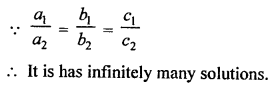Question 2.
Solution: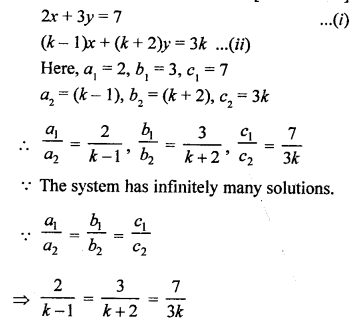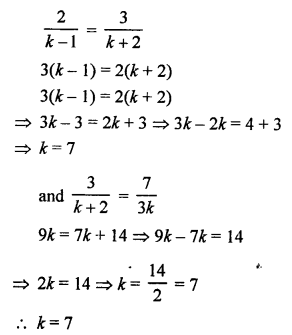Question 3.
Solution: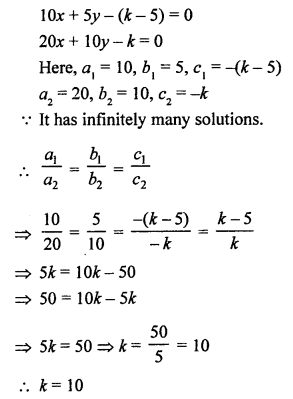Question 4.
Solution: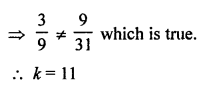Question 5.
Solution: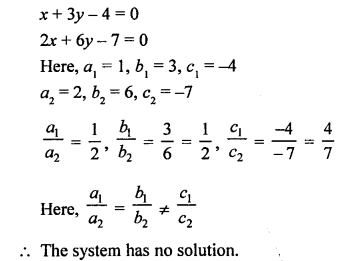Question 6.
Solution:Question 7.
Solution:
Let first, number = x
and second number = y
x – y = 5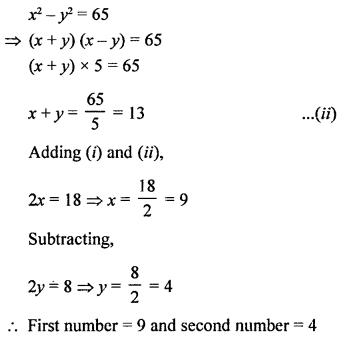Question 8.
Solution:
Let cost of one pen = ₹ x
and cost of one pencil = ₹ y
According to the conditions,
5x + 8y = 120 …(i)
8x + 5y = 153 …(ii)
13x + 13y = 273
x + y = 21 …(iii) (Dividing by 13)
and subtracting (i) from (ii),
3x – 3y = 33
⇒ x – y = 11 …….(iv) (Dividing by 3)
2x = 32 ⇒ x = 16
Subtracting,
2y = 10 ⇒ y = 5
Cost of 1 pen = ₹ 16
and cost of 1 pencil = ₹ 5

Question 9.
Solution:
Let first number = x
and second number = y
According to the conditions,and x + y = 80
⇒ x + 15 = 80
x = 80 – 15 = 65
Numbers are : 65, 15

Question 10.
Solution:
Let one’s digit of a two digits number = x
and ten’s digit = y
Number = x + 10y
By reversing its digits One’s digit = y
and ten’s digit = x
Then number = y + 10x
According to the conditions,
x + y = 10 …(i)
x + 10y – 18 = y + 10x
x+ 10y – y – 10x = 18
⇒ -9x + 9y = 18
⇒ x – y = -2 (Dividing by -9) …..(ii)
2x = 8 ⇒ x = 4
and by subtracting,
2y = 12 ⇒ y = 6
Number = x + 10y = 4 + 10 x 6 = 4 + 60 = 64

Question 11.
Solution:
Let number of stamps of 20p = x
and stamps of 25 p = y
According to the conditions,
x + y = 47 …..(i)
20x + 25y = 1000
4x + 5y = 200 …(ii)
From (i), x = 47 – y
Substituting the value of x in (ii),
4 (47 – y) + 5y = 200
188 – 4y + 5y = 200
⇒ y = 200 – 188 = 12
and x + y = 47
⇒ x + 12 = 47
⇒ x = 47 – 12 = 35
Hence, number of stamps of 20 p = 35
and number of stamps of 25 p = 12

Question 12.
Solution:
Let number of hens = x
and number of cows = y
According to the conditions,
x + y = 48 …..(i)
x x 2 + y x 4 = 140
⇒ 2x + 4y = 140
⇒ x + 2y = 70 ……(ii)
Subtracting (i) from (ii),
y = 22
and x + y = 48
⇒ x + 22 = 48
⇒ x = 48 – 22 = 26
Number of hens = 26
and number of cows = 22

Question 13.
Solution: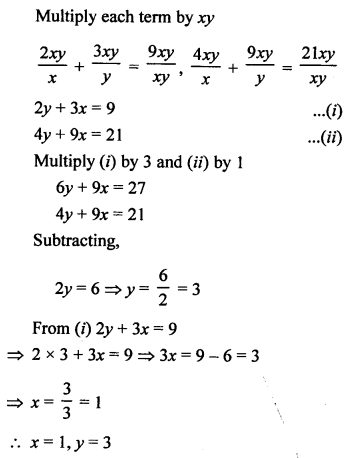Question 14.
Solution: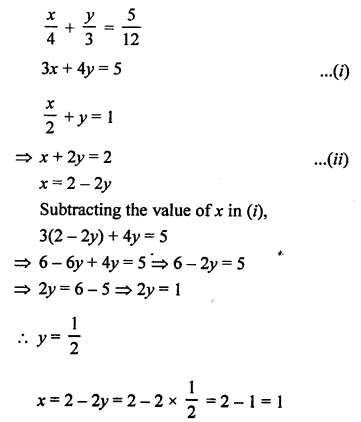Question 15.
Solution:
12x + 17y = 53 …(i)
17x + 12y = 63 …(ii)
Adding, 29x + 29y = 116
Dividing by 29,
x + y = 4 …(iii)
Subtracting,
-5x + 5y = -10
⇒ x – y = 2 …(iv) (Dividing by -5)
2x = 6 ⇒ x = 3
Subtracting,
2y = 2 ⇒ y = 1
x = 3, y = 1
x + y = 3 + 1 = 4

Question 16.
Solution: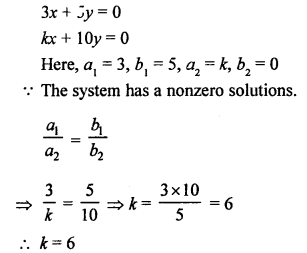Question 17.
Solution:
kx – y = 2
6x – 2y = 3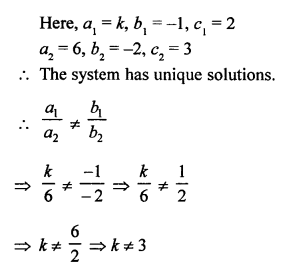Question 18.
Solution: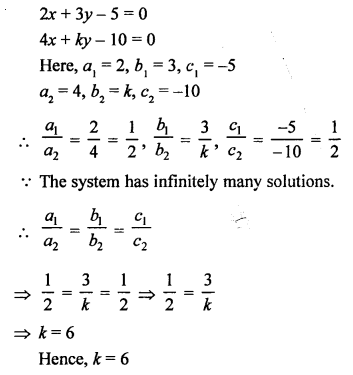Question 19.
Solution:Question 20.
Solution: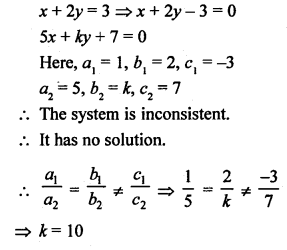Question 21.
Solution: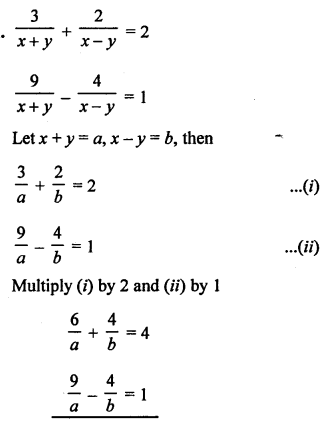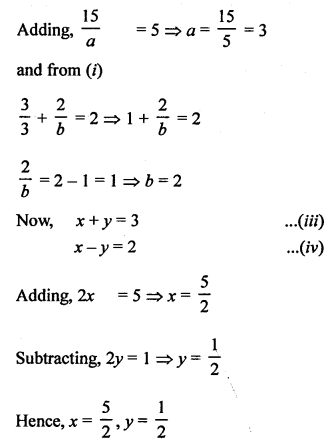Hope given RS Aggarwal Solutions Class 10 Chapter 3 Linear equations in two variables Ex 3F are helpful to complete your math homework.

If you have any doubts, please comment below. Learn Insta try to provide online math tutoring for you.